# Build a Sudoku Solver App With JavaScript

## A JavaScript app to solve sudoku puzzles using backtrackingPhoto by Richard Bell on Unsplash

Sudoku is a popular puzzle game. Humans take different approaches when solving a sudoku puzzle. But if you want a computer to solve a sudoku you have to tell a specific rule for it to follow. Backtracking is that specific technique.

If you studied Algorithms and Data Structures, you may be familiar with solving a sudoku puzzle using backtracking. You take an unsolved sudoku puzzle, solve it using backtracking, and return the solution. Today we will make a web application using JavaScript where people can input a sudoku puzzle and get the solution. This is what the completed project will look like: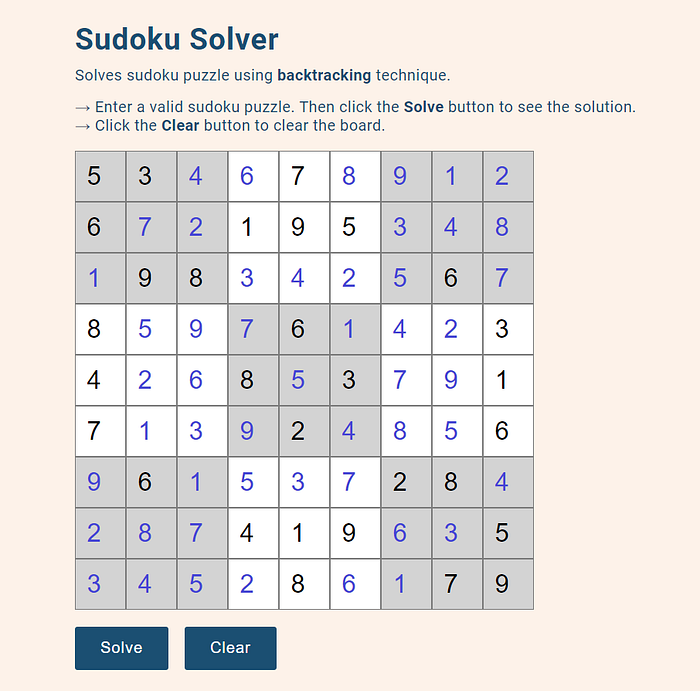Sudoku solver app

### Main Components

The main components of the project are:

• A 9x9 board. For that, we need a total of 9x9=81 input fields.
• A ‘Solve’ button. It will trigger the algorithm and solve the puzzle.
• A ‘Clear’ button to clear previous input.

### Project Layout

Here is the project structure:

``````.
├── index.html
├── scripts
│   ├── app.js
│   ├── draw_board.js
│   └── solver.js
└── styles
└── style.css
``````

`index.html` is the main HTML file. Inside the `styles` directory, the CSS files will live. We have only one CSS file, `style.css`. The `scripts` directory will contain all the JavaScript files. Instead of putting all our code in one JavaScript file, we will break down the project into three different modules:

• `draw_board.js` will draw the 81 input fields as a 9x9 sudoku board.
• `solver.js` will take the user input, solve it using backtracking, and show the result.
• `app.js` will be our main JavaScript file. Here we will import the other two modules and make the buttons work. Breaking down JavaScript code into different modules is possible because of ES6-Modules. This video helped me to understand the concept of ES6-Modules.

### Creating the Board

We start with building the sudoku board, where we can input a puzzle. Put the following code in `index.html`: The `#puzzle` div is where we will create our board. Then we have two buttons, one to solve the puzzle and one to clear the board. At this point, we have only two buttons on our page. Because we will create the input fields using JavaScript. Let’s write the following code in `scripts/draw_board.js`: We have created 81 input fields of `type=”number”` inside the `#puzzle` div: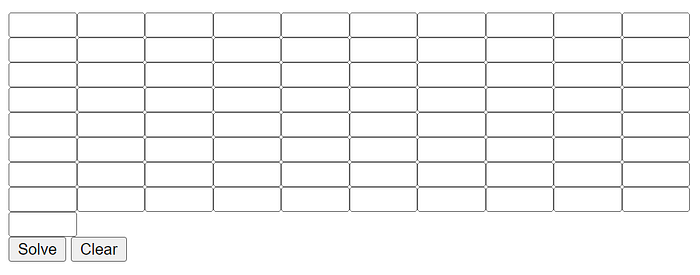Input fields are created

But we need to convert it into a 9x9 board. We can do it using CSS. Let’s do it in the `styles/style.css` file: Now the board looks like this: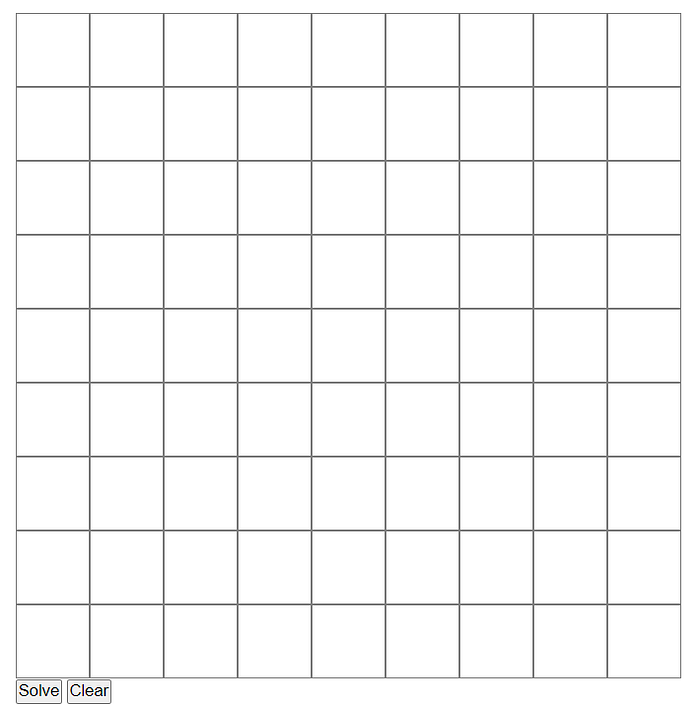Input fields converted into a 9x9 board

It would be much better if we could separate each 3x3 sub-section. We can set different colors to two consecutive 3x3 sections. For this, we need to go back to `scripts/draw_board.js` and add the following code inside the for loop: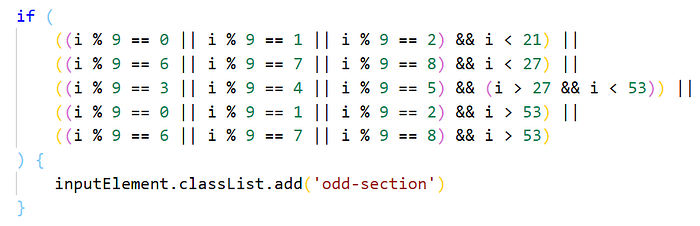The complete `draw_board.js`: This if-statement will add a `#odd-section` class to each 3x3 sub-section in the odd position. Let’s understand what I mean by odd position from the picture below: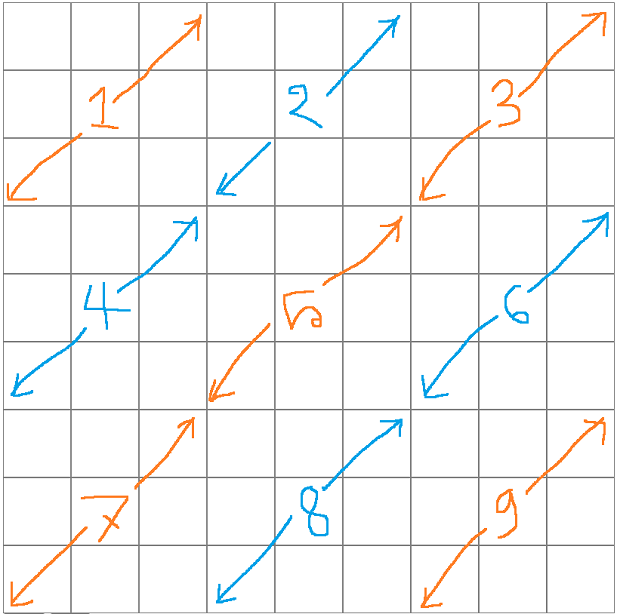Separating odd sections

Now we simply give `#odd-section` a different color in `styles/style.css`:

``````.odd-section {
background: #d3d3d3;
}
``````

The board is complete: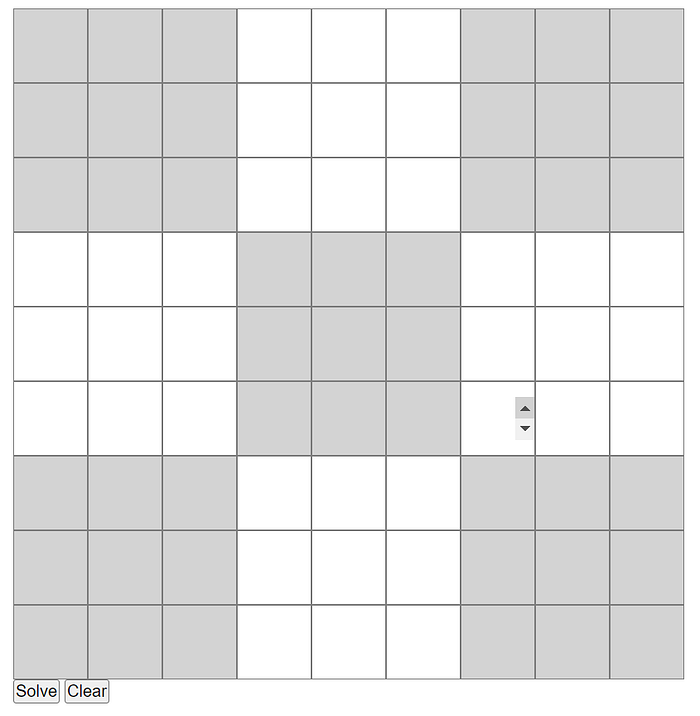The completed board

### Put User Input Into a Data Structure

First, we need to put the user input into some data structure. We will use an array for this. Let’s consider the following input: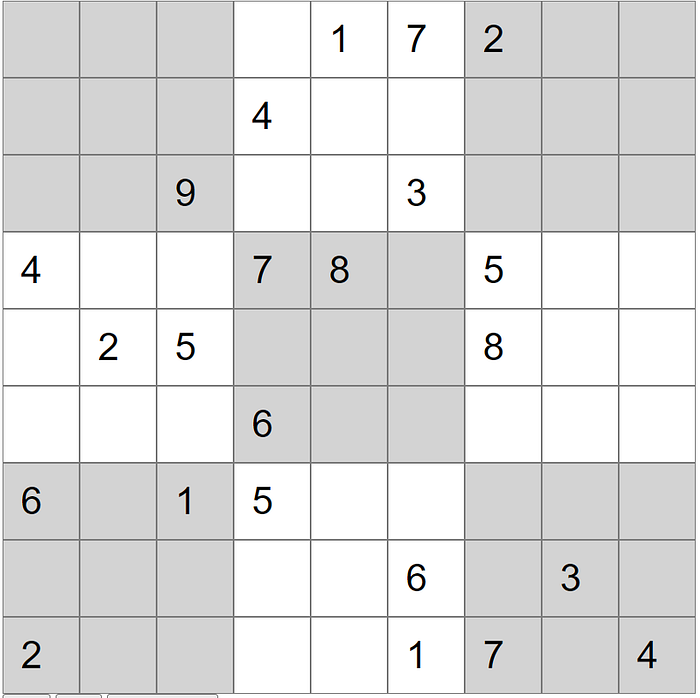An unsolved puzzle

We will take this input and put it into an array called `board`. The blank inputs will be represented by 0s in the array. The `board` array for the above input will be this: We need to write some logic so that our code understands the blank inputs and put the values properly in the array. Let’s go to `scripts/solver.js `and put this code: Here we are querying all the input fields. If there is a value we put the value in the array. If there is no value, that means empty input, we put a 0 in the array. Now that we have an array containing an unsolved puzzle, it’s time to solve it!

### Solving the Puzzle

We will solve the puzzle using backtracking. After solving the puzzle the `board` array will contain the solution. I wrote an article on how the backtracking technique solves sudoku. You can read it to have a better understanding of what we are doing here: In this article, I used a two-dimensional array to solve the puzzle. But for this project, I decided to use a one-dimensional array because it makes getting the input and showing the result back much easier. Here is the blog post from where I learned to do it using a 1-d array: Add the following code to `scripts/solver.js`: Now if we run the `solve()` function, the `board` array will have the solution to the puzzle. We need to display the solution back on our website. Add this function to `scripts/solver.js`: This function will populate the input fields with the elements of the `board` array. We have our solution! Most of the work is done. Now we just need to put all the small pieces of code together and complete the `scripts/app.js` file.

### Complete the Code

In `scripts/app.js` we will import the other two modules: `dreaw_board.js` and `solver.js`. And we will make the ‘Solve’ button work and the ‘Clear’ button work. Here is the complete `app.js`: Now if we input a puzzle and hit ‘Solve’ we will get the solution. For the ‘Clear’ button, we will simply reload the browser similarly we refresh any page using the refresh button on our browser. This will remove previous inputs and clear the board for new input.

### Final Touch

Finally, we will add some text to the HTML and some styling to the page. Complete `index.html`: Complete `style.css`: The project is complete! Now we have an app that solves sudoku puzzles for us.

### Find the Project

You can find the source code of this project from the GitHub repository: Use the code to make your own version and feel free to give any suggestions to make it better. Check the live demo here:

### Conclusion

By building this project we learned some valuable things. We learned how to turn an algorithmic problem into a web application that people can actually use. We learned how we can modularize our JavaScript code with the help of ES6-Modules. I hope you find it helpful. Thanks for reading.

### Resources

• https://lisperator.net/blog/javascript-sudoku-solver
• https://www.tutorialspoint.com/es6/es6%5Fmodules.htm
• https://python.plainenglish.io/solve-a-sudoku-puzzle-using-backtracking-in-python-8e9eb58e57e6